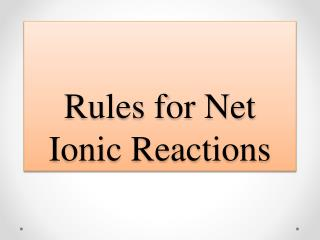DownloadDownload PresentationRules for Net Ionic Reactions

# Rules for Net Ionic Reactions

Télécharger la présentation## Rules for Net Ionic Reactions

- - - - - - - - - - - - - - - - - - - - - - - - - - - E N D - - - - - - - - - - - - - - - - - - - - - - - - - - -
##### Presentation Transcript

1. Rules for Net Ionic Reactions

2. Background: valences and formulas •Charge can also be found via the compound •E.g. in NaNO3(aq) if you know Na forms Na+, then NO3must be NO3–(NaNO3is neutral) •By knowing the valence of one element you can often determine the other valences Q -Write the ions that form from Al2(SO4)3(aq)? Step 1-look at the formula: Al2(SO4)3(aq) Step 2-determine valences: Al3 (SO4)2 (Al is 3+ according to the periodic table) Step 3-write ions: 2Al3+(aq) + 3SO42–(aq) •Note that there are 2 aluminums because Al has a subscript of 2 in the original formula

3. Review: forming ions • Ionic (i.e. salt) refers to +ve ion plus –ve ion. • Usually this is a metal + non-metal or metal + polyatomic ion (e.g. NaCl, NaClO3, Li2CO3) • Polyatomic ions are listed on the web site, • (aq) means aqueous (dissolved in water) • For salts (aq) means the salt exists as ions • NaCl(aq) is the same as: Na+(aq) + Cl–(aq) • Acids form ions: HCl(aq) is H+(aq) + Cl–(aq), • Bases form ions: NaOH(aq) is Na++ OH– Q -how is charge determined (+1, -1, +2, etc.)? • F, Cl electron, thus forming • Caelectrons, thus forming

4. Practice with writing ions • Q -Write ions for Na2CO3(aq) • •Notice that when ions form from molecules, charge can be separated, but the total charge (and number of each atom) stays constant. • Q -Write ions for Ca3(PO4)2(aq) & Cd(NO3)2(aq) • Q -Write ions for Na2S(aq) and Mg3(BO3)2(aq)

5. Writing Types of Chemical Equations • Written equations can be divided into 3 types 1) Molecular, 2) Ionic, 3) Net ionic • •Here is a typical molecular equation: • Cd(NO3)2(aq) + Na2S(aq) →CdS(s) + 2NaNO3(aq) • •We can write this as an ionic equation (all compounds that are (aq) are written as ions): • Cd2+(aq) + 2NO3–(aq) + 2Na+(aq) + S2–(aq) →CdS(s) + 2Na+(aq) + 2NO3–(aq) • •To get the NET ionic equation we cancel out all terms that appear on both sides:Net: Cd2+(aq) + S2–(aq) →CdS(s)

6. Equations must be balanced • There are two conditions for molecular, ionic, and net ionic equations: • Materials balance • Both sides of an equation should have the same number of each type of atom • Electrical balance • Both sides of a reaction should have the same net charge

7. Q-When NaOH(aq) and MgCl2(aq) are mixed, _______(s) and NaCl(aq) are produced.Write balanced molecular, ionic & net ionic equations • First write the skeleton equation • NaOH(aq) + MgCl2(aq) →Mg(OH)2(s) + NaCl(aq) • Next, balance the equation Ionic equation: • 2Na+(aq) + 2OH-(aq) + Mg2+(aq) + 2Cl-(aq) →Mg(OH)2(s) + 2Na+(aq) + 2Cl-(aq) • Net ionic equation: • 2OH-(aq) + Mg2+(aq) →Mg(OH)2(s) • Write balanced ionic and net ionic equations: • CuSO4(aq) + BaCl2(aq) →CuCl2(aq) + BaSO4(s) • Fe(NO3)3(aq) + LiOH(aq) →______(aq) + Fe(OH)3(s) • Na3PO4(aq) + CaCl2(aq) →_________(s) + NaCl(aq) • Na2S(aq) + AgC2H3O2(aq) →________(aq) + Ag2S(s)

8. Solubility • Precipitation refers to the formation of a solid from ions. A precipitate is “insoluble” • Soluble and insoluble are general terms to describe how much of a solid dissolves. • Solubility can be predicted from rules • These are general rules, based on observation • To determine solubility, follow the rules in order • You will not have to memorize these rules (yet), you will have to use the rules to predict solubility